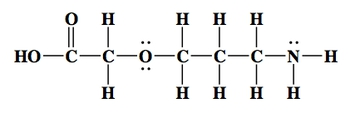## General Chemistry

Learn the toughest concepts covered in Chemistry with step-by-step video tutorials and practice problems by world-class tutors

22. Organic Chemistry

# Structural Formula

An organic compound can be written in different ways. Each one is a unique expression of the bonds each element is making.

Molecular Formulas
1
concept

## Structural Formula vs6m
Play a video:
Hey, guys, In this new video, we're gonna take a look at structural formulas. So we're gonna say your typical organic molecule can be drawn in a few different ways. So if we take, for example, painting paintings formula is C five h 12 Now there are different ways we can draw it. The three major ways that we can talk about it is our structural formula in our structural formula. We show all the connections that each of the elements is making. We also call this our expanded formula expanded because we've blown it out where every single bond is shown. And remember, carbon is Tetra Valent, Tetra valent, meaning that carbon wants to make four bonds when it's neutral. So here, if you take a look, each carbon in the center is making four bonds in some way, either by making those bonds with hydrogen or with carbons around them. They're all making four bonds Now, here you can transition away from your structural formula toe what's called your condensed formula. So you're condensed formula. You basically just push the bonds back in around that central element. So if you take a look, this carbon here it is connected to 123 hydrogen. So it's a CH three group. So there goes our stage three groups, this carbon. Here it is connected toe 12 carbons. So it's a CH two group. This carbon here is connected to 12 It's also ch two. This carbon here is connected toe 12 It is also a ch two. And then finally, the carbon at the end is connected. Toe 123 hydrogen. That's why it's a CH three. So we go from the structural or expanded formula to the condensed formula when we push the bonds inward so everything's compact, basically, and then next we move to the skeletal formula. When you guys get to organic, you'll also hear being called by different names. We can hear that it's called by a new name, which is called the Cuckoo Lee, or Cake. You'll structure So K E K U l e. With a little, uh, accent mark above the so called Coulier or cake You'll, depending on which professor you get because they pronounced him differently. Um, that's a structural formula. Skeletal formula. Now, how do we read something like this? What you need to realize here is that every end is a carbon every end and every corner is a carbon. So there's a carbon here, here, here, here and here. So at both ends there's a carbon, and basically at every edge there's a carbon. Now the rule about skeletal formula is that you cannot show carbons. Carbons are invisible, they're there, and we just can't draw them. And also, any hydrogen is connected to them are also invisible, so the carbons are invisible and the hydrogen is connected to them are invisible. Now, if you have an O. H or N H or some other group, if you have a different element than carbon and it has a cheese on it, then you'd have to show that different element, and you can show the hydrogen is connected to that different element. So again, when it comes to our skeletal formula, carbons are invisible and the hydrogen is connected to them are also invisible. The Onley way I could show those hydrogen is that those hydrogen were connected to something different from carbon, such as a halogen, such a sulfur or phosphorus or oxygen or nitrogen. Now, remember, we said that carbon is tetro Valent, meaning it needs to make four bonds to be satisfied. So every end, every corner is a carbon. So what you need to realize here is that if this is a carbon, we see it making one bond with another carbon. So that must mean it has three hydrogen is on it that we don't see. Why does it need three hydrogen? Because again, carbon is tetra valid. It needs to make four bonds, then hear this. Carbon is, um we see it making one bond to bonds. So that means it has to hydrogen is we don't see toe. Help it get to four. Bonds totaled. Same thing with this carbon. We see it's connected toe 12 carbons, so it must have to hydrogen is we don't see in order to get to four here, this carbons making one to bond. So it needs to more hydrogen to get to four. And then finally the end carbon. We see him making one bond here, so I must have three hydrogen. We don't see now. You're also hear about your professor talking about the ball and stick way of drawing these. That's with, like, models and stuff like that. But when it comes to actually taking the exam and doing the work, um, either online or in class. These are the three predominant formulas that we use for any organic molecule we can draw as a structural formula where we expand all the bonds out. We can draw it as a condensed formula where we push the bonds in, or we can draw it as a skeletal formula. What we do not show any carbons or the hydrogen is connected to them. Now that we've seen this, I want you guys to attempt to do some of these example questions. We'll come back, take a look at each 11 by one and see what's the best way to look at. Remember every corner, every edge is a carbon. So I hope you guys a little bit on this first one. So every end, every edge is a carbon. So all those that have marked our carbons and remember carbon is Tetra Valent. So what does Tetra Valent mean? Use that to determine the number of hydrogen on each of those carbons. Once you figure out the total number of elements, then you can get the molecular formula also remember here, Why do I have to show this hydrogen now? Because it's connected to an element different from carbon. It's connected to an oxygen. So now we can show it. This oxygen up here is different from carbon. It has no hydrogen on it. We know that hydrogen is not gonna be invisible on it because again, if it's an element different from carbon, we can show the hydrogen is connected to it. So guys, try to attempt to do this question on your own will come back and take a look at this first one here.

In the structural formula all the individual bonds are shown. This representation is sometimes called the expanded chemical formula.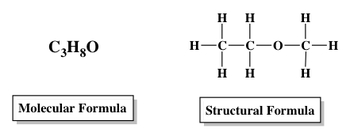In the condensed formula the bonds are pushed in to give a more compact representation of the bonds.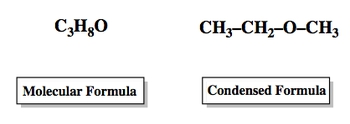In the skeletal formula all carbons and the hydrogens connected to them are not shown. Only elements different from carbon and the hydrogens connected to them can be represented.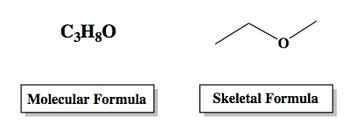2
example

## Determine the molecular formula for the following organic compound1m
Play a video:
Alright, guys. So let's look at this example here. So every corner, every edge is a carbon, right? So we know that carbon must make four bonds this carbon. Here we see it making one bond to that carbon. So it must have three inches on it to get to four. This carbon here we said, making 1234 bonds. So it has normal room to accept any hydrogen. So it's fine. This is a carbon here. We see making one bond. So it must have three h is that we don't see to get it to four. Same with this carbon. We see him making one bond, so it must have three hydrogen on it that we don't see. This carbon here is making to bonds 12 So it must have to hydrogen is we don't see. And then this carbon here is making 1234 bonds total. So it's satisfied in terms of being Tetra Valent has no hydrogen on it at all. So let's count how many total carbons we have. We have 123456 carbon. So C six next hydrogen is We have three, 69 11 12. And then how Maney Oxygen's do we have? We have to oxygen's. So this will be your molecular formula C six h 12 02 That would be the former that you should have gotten for this first compound here. Now that we've done that, one try to do this one. This one isn't written as a skeletal formula. I've shown it as an expanded or structural formula. So this one should be a lot easier. Just literally count the number of elements and then give me the structural formula for it. Come back. Let's take a look at it once you've done that.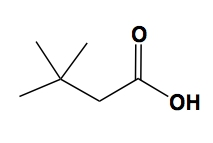3
example

## Convert the following structural formula into its condensed formula1m
Play a video:
Hey, guys. So here we have to convert the following structural formula into its condensed formula. So basically, we just have to pack things in, and we're gonna say to do this and we're gonna take a look at this skeleton here, this carbon skeleton, and we're just gonna compress all the either hydrogen or ch three groups onto them. So we have. We'll say carbon 12345 and six. So the first carbon carbon one has on it. Three hydrogen, So compress it all into ch three. Then this carbon, the second carbon has to hydrogen on it. That ch two, then the third carbon this carbon has on it. What? Ah, hydrogen. And another ch three group. We're gonna put hydrogen and then to say that ch through ch three group is branching off. We put it in parentheses. We don't have to do that for the hydrogen because they can just pack in like this with it. But with other groups, we have to put in parentheses. Then we have next this carbon carbon four, which has on it two hydrogen. Then we have carbon five, which has one hydrogen. Now we draw the double bond. And then this last carbon carbon six has on it to hydrogen, so that would represent are condensed formula. So we've gone from the expanded structural formula tome or condensed formula, basically just taking the surrounding hydrogen, packing them in more tightly, take that ch three group packet and more tightly to get our condensed formula.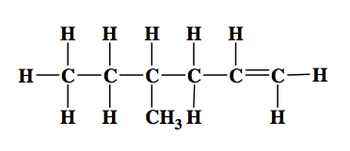4
example

## Determine the molecular formula for the following organic compound1m
Play a video:
Hey, guys. So here we have to determine the molecular formula for the following organic compound. So here we have to count correctly the number off each element. Remember every ending corners of carbon. So each one of these represents a carbon. Okay, so we're gonna say each of them is a carbon. So let's actually draw that carbon in. Remember, carbon must make four bonds, so each one of those carbons. So this carbon here we see making 123 bonds. So it has one hydrogen. This one's already making 1234 bonds. So it's good. This one has made you 1234 bonds. So it's good. This one's only making three. So it has one hydrogen and sing with these other two. Now, if we're gonna count, let's see how many carbons we have. So we have one, 234567 carbons, C seven. Hydrogen is how many hydrogen do we have? And then how maney Oxygen's do we have? We have to hear. Plus, another one is three. So that's +03 So the formula here a molecular formula ABC, 78603 Again, we're only covering the simplest part of organic chemistry. When you move on to the actual organic chemistry, you'll be dealing with much larger structures, but it's always important, established the fundamentals like we're doing here.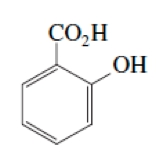5
example

## Determine the molecular formula for the following organic compound1m
Play a video:
Alright, guys. So you guys enough time to take a look at this. Remember, every edge, every end is a carbon. So there's a carbon here, here, here and here. Carbon must make four bonds. This one here is only making three bonds that we see. So should have had one h and same with this one. The other two are already making four bonds they don't have. They have no hydrogen at all. So if we take a look, we say the formula is there are four carbons will see four and he really doesn't matter the order, Um, in terms of the number of elements because we're just learning how to read these structures now. So all that's important is getting the right number of each element. So here we have four carbons. Let's move on to hydrogen is next. So we have 1234 Hydrogen nitrogen is typically listed next and oxygen last. So nitrogen is here. It has won two nitrogen and then to oxygen's. So this should have been the formula that you got C four h four and Make sure you go back. If you didn't get this correct and see what exactly might have gone wrong. Remember every edge. Every end is a carbon count the number of bonds that each carbon is making to determine if they have any hydrogen zit. All carbon is Tetra Valent and wants to make four bonds when it's neutral. So if you see making less than four, that's because it has hydrogen czar invisible, and you have to figure out how many it has. So we've done these two together. Let's see if you guys can attempt to do the last example one here on the bottom of the page, come back and see how best I answer this next question.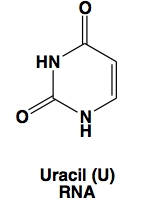6
example

## Convert the following structural formula into the carbon skeleton formula, otherwise known as a kekulé structure1m
Play a video: